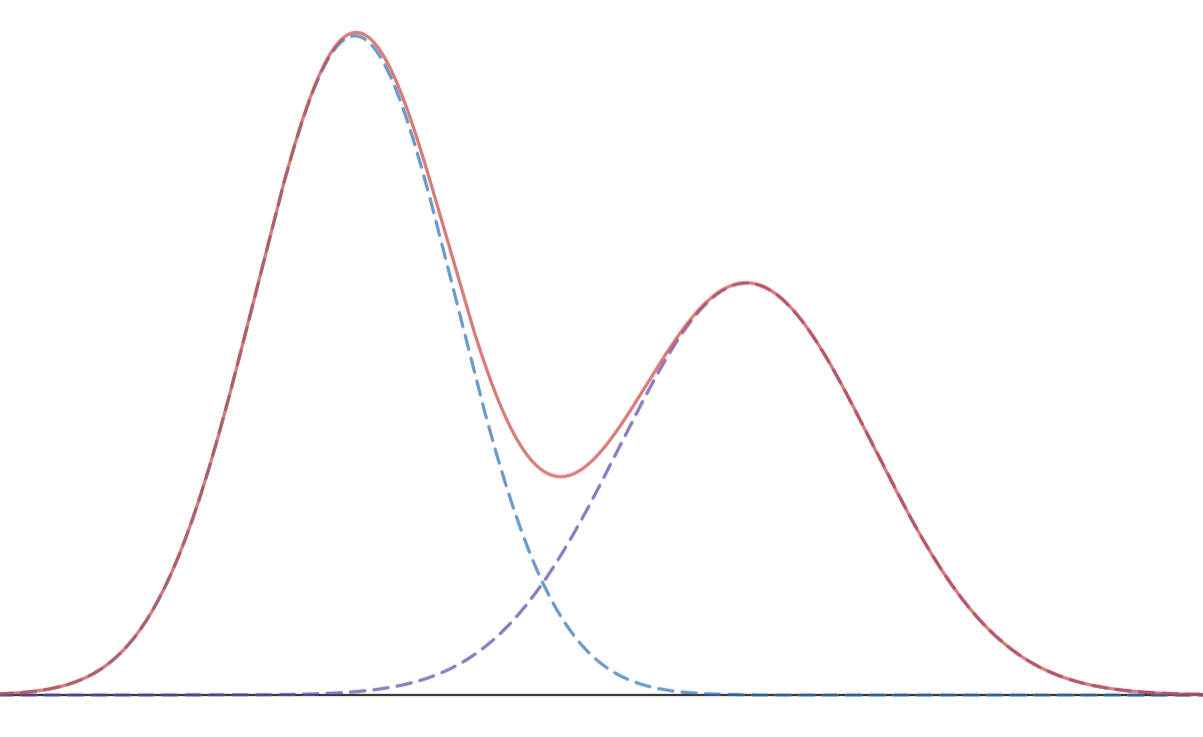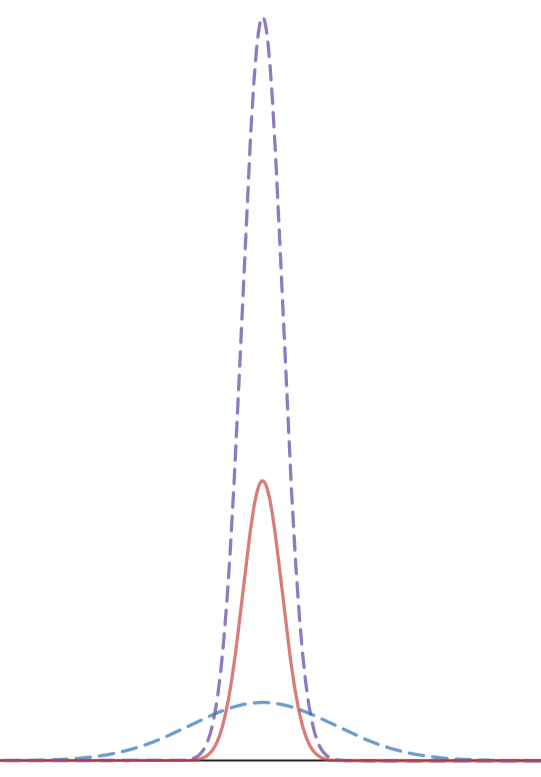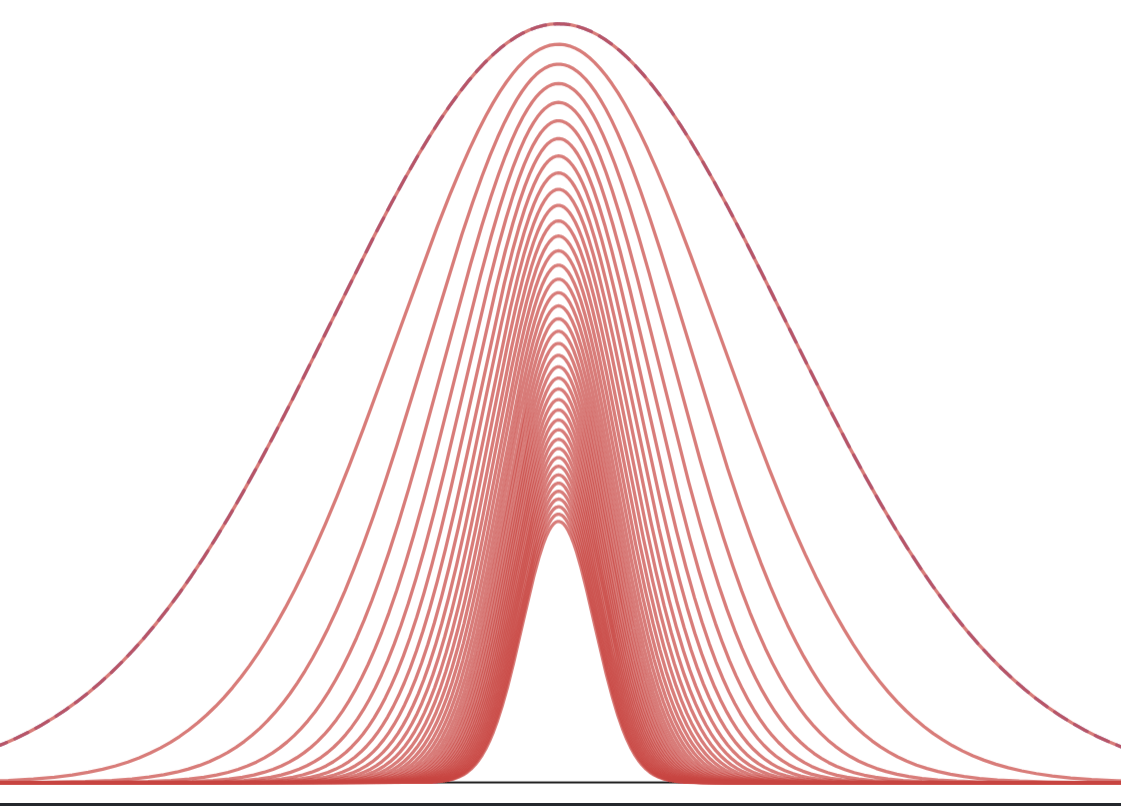# Gaussians are making Gaussians

Let$f$ and$g$ be Gaussian distributions.Well, of course not. They don’t have the same mean. So set the means equal.That’s no better. The sum of$f$ and$g$ is still very much not Gaussian.So, that’s no good. But of course it failed — just look at those visuals!

What about multiplication? Here’s what the product of two Gaussian distributions with equal means looks like.That looks much better!

In fact this is true: the product of two Gaussians distributions remains a Gaussian function. The only proofs I know of dive into some algebra — I like this one — but the core idea is that multiplying exponents is additive. That’s what keeps it all in the Gaussian family.

So consider two Gaussian functions, one with a mean$\mu$ and the other with a mean at 0 (for a touch of simplicity):$f(x) = \frac{1}{\sqrt{2\pi}\sigma_f} e^{\frac{x^2}{2\sigma^2_f}}$$g(x) = \frac{1}{\sqrt{2\pi}\sigma_g} e^{\frac{(x -\mu)^2}{2\sigma^2_g}}$

Their product will look like this:$f(x)g(x) = \frac{1}{2\pi\sigma_f\sigma_g} e^{\frac{x^2}{2\sigma^2_f}+\frac{(x-\mu)^2}{2\sigma^2_g}}$

Making common denominators and adding through:$f(x)g(x) = \frac{1}{2\pi\sigma_f\sigma_g} e^{\frac{\sigma^2_g x^2 +\sigma^2_f(x -\mu)^2}{2\sigma^2_f\sigma^2_g}}$

Might as well expand that exponent a bit and summarize:$f(x)g(x) = \frac{1}{2\pi\sigma_f\sigma_g} e^{\frac{(\sigma^2_f +\sigma^2_g) x^2 -2\sigma^2_f x \mu + \sigma^2_f \mu^2}{2\sigma^2_f\sigma^2_g}}$

And then you can divide the numerator and denominator by$(\sigma^2_f +\sigma^2_g)$ and you’ll end up with a quadratic trinomial in$x$. You can always express that quadratic trinomial as$(x - M)^2$ somehow or another.

(Brief but important nit-picky note: that would make the product of two Gaussian function, but the scale factor on the left side of the expression is off, so it’s not a Gaussian distribution. You’d have to scale the product of two Gaussian distribution in order to get another Gaussian distribution.)

Is this useful? Is this significant, in some way? I don’t know. Apparently it’s useful in applying Bayes’ Theorem, but I know nothing about that.

One thing I do know is that it makes for some fun visuals.## One thought on “Gaussians are making Gaussians”

1.David Wees says:

This made me wonder if the set of Gaussian distributions under multiplication form a group. Is there an identity Gaussian e that doesn’t change any other distribution when multiplied by it? For any Gaussian distribution f, is there another Gaussian distribution g such that f × g = e? It looks like multiplication of Gaussian distributions is commutative and associative.